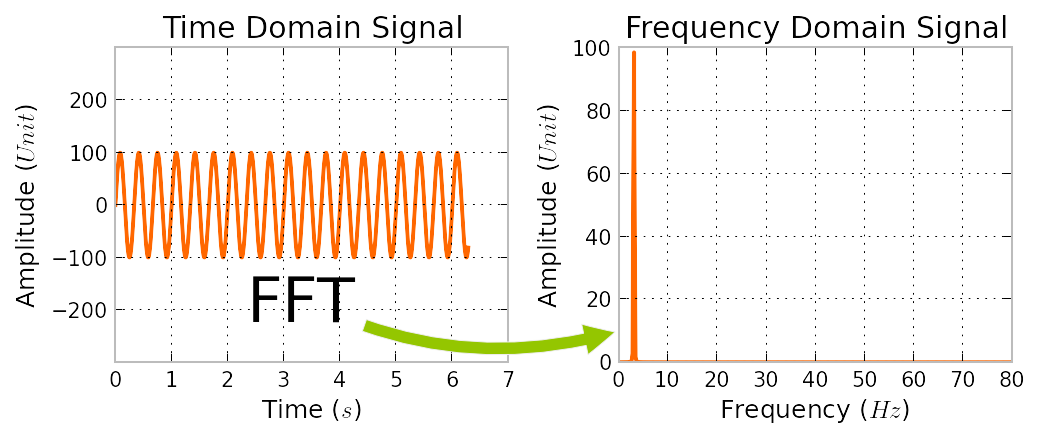﻿ WHAT IS FFT AND DFT?Back

## WHAT IS FFT AND DFT?FFT : Fast Fourier Transform. The FFT is a faster version of the DiscreteFourier Transform (DFT). The FFT utilizes some clever algorithms to do the same thing as theDTF, but in much less time.DFT:       Iis extremely important in the area of frequency (spectrum)analysis because it takes a discrete signal in the time domain and transforms that signal into itsdiscrete frequency domain representation. Without a discrete-time to discrete-frequency transformwe would not be able to compute the Fourier transform with a microprocessor or DSP based system.It is the speed and discrete nature of the FFT that allows us to analyze a signal’s spectrum with Matlab